Custom SearchHIGH-PASS FILTER A high-pass filter circuit passes all currents having a frequency higher than a specified frequency, while opposing all currents having a frequency lower than its specified frequency. This is illustrated in figure 1-17. A capacitor that is used in series with the source of both high and low frequencies, as shown in view (A) of figure 1-18, will respond differently to high-frequency, low-frequency, and direct currents. It will offer little opposition to the passage of high-frequency currents, great opposition to the passage of low-frequency currents, and completely block direct currents. The value of the capacitor must be chosen so that it allows the passage of all currents having frequencies above the desired value, and opposes those having frequencies below the desired value. Then, in order to shunt the undesired low-frequency currents back to the source, an inductor is used, as shown in view (B). This inductor must have a value that will allow it to pass currents having frequencies below the frequency cutoff point, and reject currents having frequencies above the frequency cutoff point, thus forcing them to pass through the capacitor. By combining inductance and capacitance, as shown in view (C), you obtain the simplest type of high-pass filter. At point P most of the high-frequency energy is passed on to the load by the capacitor, and most of the low-frequency energy is shunted back to the source through the inductor. Figure 1-17. - High-pass filter response curve.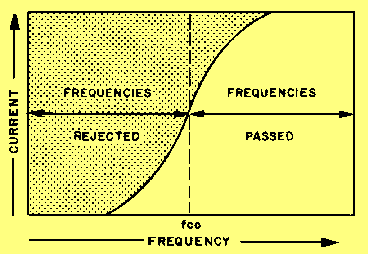Figure 1-18A. - Components of a simple high-pass filter.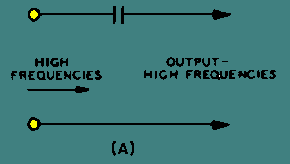Figure 1-18B. - Components of a simple high-pass filter.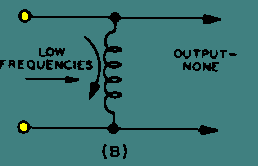Figure 1-18C. - Components of a simple high-pass filter.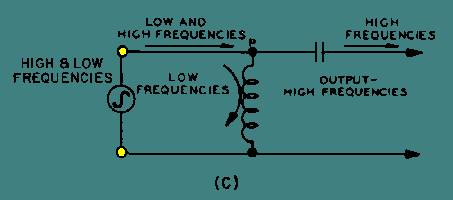Resonant circuits can be made to serve as filters in a manner similar to the action of individual capacitors and inductors. As you know, the series-LC circuit offers minimum opposition to currents that have frequencies at or near the resonant frequency, and maximum opposition to currents of all other frequencies. You also know that a parallel-LC circuit offers a very high impedance to currents that have frequencies at or near the resonant frequency, and a relatively low impedance to currents of all other frequencies. If you use these two basic concepts, the BANDPASS and BAND-REJECT filters can be constructed. The bandpass filter and the band-reject filter are two common types of filters that use resonant circuits. Bandpass Filter A bandpass filter passes a narrow band of frequencies through a circuit and attenuates all other frequencies that are higher or lower than the desired band of frequencies. This is shown in figure 1-19 where the greatest current exists at the center frequency (fr). Frequencies below resonance (f1) and frequencies above resonance (f2) drop off rapidly and are rejected. Figure 1-19. - Bandpass filter response curve.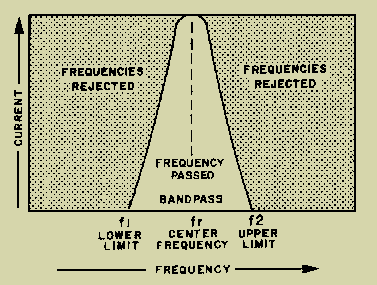In the circuit of figure 1-20, view (A), the series-LC circuit replaces the inductor of figure 1-16 , view (A), and acts as a BANDPASS filter. It passes currents having frequencies at or near its resonant frequency, and opposes the passage of all currents having frequencies outside this band. Figure 1-20A. - Components of a simple bandpass filter.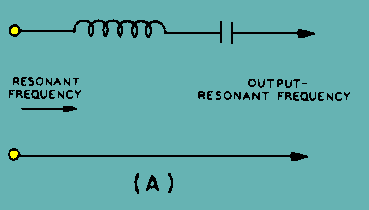Thus, in the circuit of figure 1-20, view (B), the parallel-LC circuit replaces the capacitor of figure 1-16, view (B). If this circuit is tuned to the same frequency as the series-LC circuit, it will provide a path for all currents having frequencies outside the limits of the frequency band passed by the series-resonant circuit. The simplest type of bandpass filter is formed by connecting the two LC circuits as shown in figure 1-20, view (C ). The upper and lower frequency limits of the filter action are filter cutoff points. Figure 1-20B. - Components of a simple bandpass filter.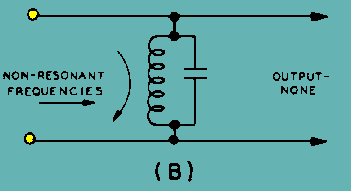Figure 1-20C. - Components of a simple bandpass filter.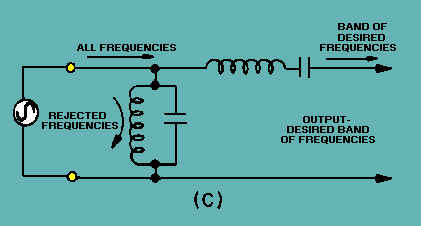Band-Reject Filter A band-reject filter circuit is used to block the passage of current for a narrow band of frequencies, while allowing current to flow at all frequencies above or below this band. This type of filter is also known as a BAND-SUPPRESSION or BAND-STOP filter. The way it responds is shown by the response curve of figure 1-21. Since the purpose of the band-reject filter is directly opposite to that of a bandpass filter, the relative positions of the resonant circuits in the filter are interchanged. The parallel-LC circuit shown in figure 1-22, view (A), replaces the capacitor of figure 1-18, view (A). It acts as a band-reject filter, blocking the passage of currents having frequencies at or near resonant frequency and passing all currents having frequencies outside this band. The series-LC circuit shown in figure 1-22, view (B), replaces the inductor of figure 1-18, view (B). If this series circuit is tuned, to the same frequency as the parallel circuit, it acts as a bypass for the band of rejected frequencies. Then, the simplest type of band-reject filter is obtained by connecting the two circuits as shown in figure 1-22, view (C ). Figure 1-21. - Band-reject filter response curve.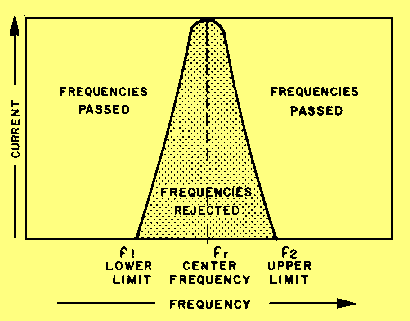Figure 1-22A. - Components of a simple band-reject filter.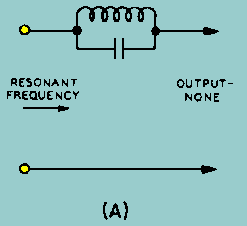Figure 1-22B. - Components of a simple band-reject filter.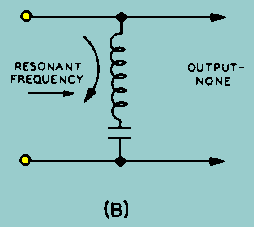Figure 1-22C. - Components of a simple band-reject filter.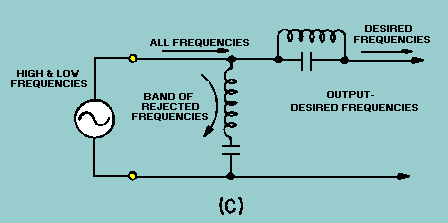Q.14 What is the device called that will separate alternating current from direct current, or that will separate alternating current of one frequency from other alternating currents of different frequencies?Q.15 What are the four general types of filters?Q.16 What is the filter called in which the low frequencies do not produce a useful voltage?Q.17 What is the filter called that passes low frequencies but rejects or attenuates high frequencies?Q.18 How does a capacitor and an inductor react to (a) low frequency and (b) high frequency?Q.19 What term is used to describe the frequency at which the filter circuit changes from the point of rejecting the unwanted frequencies to the point of passing the desired frequencies?Q.20 What type filter is used to allow a narrow band of frequencies to pass through a circuit and attenuate all other frequencies above or below the desired band?Q.21 What type filter is used to block the passage of current for a narrow band of frequencies, while allowing current to flow at all frequencies above or below this band?Integrated Publishing, Inc. - A (SDVOSB) Service Disabled Veteran Owned Small Business